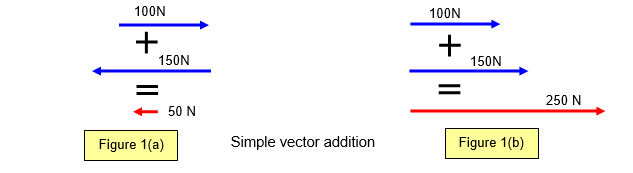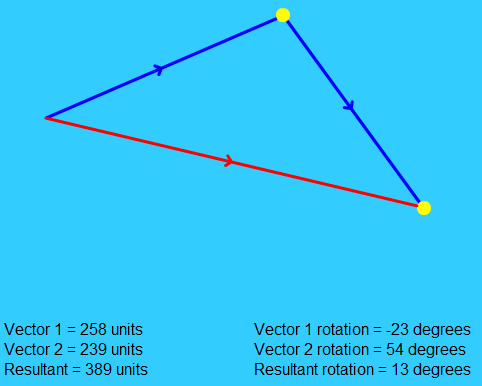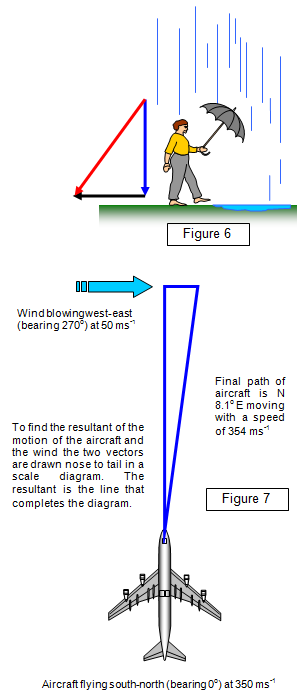When two or more vectors are added the resulting sum of the vectors is called the RESULTANT vector or simply the RESULTANT. This could be a resultant velocity, force, acceleration etc. depending on the nature of the original vectors.

We will consider the addition of two or more vectors first when they all act in the same line and then when they act at angles to each other.

(i) vectors acting in the same line
Two or more vectors acting in the same direction may be added as if they were scalars. For example the sum, or resultant of the three forces shown in Fig. 1(a) is 50 N acting right to left while in (b) it is 250 N left to right.(ii) vectors acting in different directions
However if the two vectors are not acting along the same line the triangle of vectors shown below can be used to add them together.Using the same magnitude for the two vectors as those already considered we draw a scale diagram in both magnitude and direction as shown in Figure 2. The resultant (R) (= 214N in this case) is the vector that closes the triangle.

Notice that the original two vectors (shown blue in the diagram) follow each other round the triangle (nose to tail) to give the resultant, the red vector (R), and that this resultant acts in the opposite direction round the triangle.

If the two vectors act at 90o to each other then the resultant can be found from the following diagrams (Figures 3(a) and (b).The two vectors (A and B) that you wish to add are represented in magnitude and direction by a scale diagram as before (see Figure 2). In this example A = 400 N, B = 300 N and the resultant (R) is then found by measuring the closing vector and is found to be 500 N.The resultant of the two vectors can also be found by calculation.
Resultant (R) = ( 4002 + 3002)1/2 = (25000)1/2 = 500 N
The direction of R can be found from: tan θ = 300/400 = 0.75 and so θ = 36.9o.For the schoolphysics animation showing the addition of vectors please click the symbol:

An example of a screen from this animation is shown below.If more than two vectors act at a point as in Figure 5(a) then the polygon of vectors can be used. The resultant is still the vector (shown red) that closes the polygon. (See Figure 5(b)).

The original vectors follow nose to tail around the polygon while the resultant faces the opposite way.### Walking in the rain – vector addition

A man walking through a rainstorm is a good example of the addition of vectors (see Figure 6).

If he walks at 1.5 ms-1 and the rain is falling vertically at 2 ms-1 then the rain will be hitting him at 2.5 ms-1 at an angle of 37o to the vertical.

### Aircraft and a cross wind

The next example of vector addition shows an aircraft flying on an initial bearing of 0o at 350 ms-1 with a cross wind blowing west-east at 50 ms-1. (See Figure 7)

The resulting velocity of the plane relative to the ground is 354 ms-1 on a bearing of N 8.1o E.

A VERSION IN WORD IS AVAILABLE ON THE SCHOOLPHYSICS USB